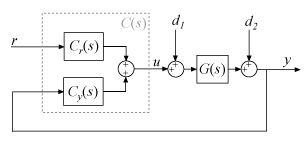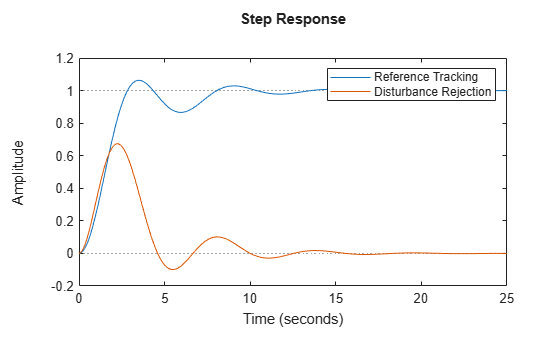Documentation

### This is machine translation

Mouseover text to see original. Click the button below to return to the English version of the page.

Note: This page has been translated by MathWorks. Click here to see
To view all translated materials including this page, select Country from the country navigator on the bottom of this page.

# getPIDLoopResponse

Closed-loop and open-loop responses of systems with PID controllers

## Syntax

``response = getPIDLoopResponse(C,G,looptype)``

## Description

example

````response = getPIDLoopResponse(C,G,looptype)` returns a response of the control loop formed by the PID controller `C` and the plant `G`. The function returns the closed-loop, open-loop, controller action, or disturbance response that you specify with the `looptype` argument. The function assumes the following control architecture.When `C` is a `pid` or `pidstd` controller object (1-DOF controller):When `C` is a `pid2` or `pidstd2` controller object (2-DOF controller):```

## Examples

collapse all

Design a PI controller for a SISO plant and examine its performance in reference tracking and disturbance rejection. For reference tracking, use the `"Closed Loop"` response. For rejection of a load disturbance, use `"Input Disturbance"`.

```G = tf(1,[1 1 1]); C = pidtune(G,'PI'); Tref = getPIDLoopResponse(C,G,"Closed Loop"); Tdist = getPIDLoopResponse(C,G,"Input Disturbance"); step(Tref,Tdist) legend("Reference Tracking","Disturbance Rejection")```Validate the tuned controller by comparing the extracted responses to your design requirements for settling time and overshoot.

Design a two-degree-of-freedom (2-DOF) PID controller for a plant and examine its performance in reference tracking and disturbance rejection. For reference tracking, use the `"Closed Loop"` response. For rejection of a load disturbance, use `"Input Disturbance"`.

```G = tf(1,[1 0.5 0.1]); w0 = 1.5; C = pidtune(G,'PID2',w0); Tref = getPIDLoopResponse(C,G,"Closed Loop"); Tdist = getPIDLoopResponse(C,G,"Input Disturbance"); step(Tref,Tdist) legend("Reference Tracking","Disturbance Rejection")```## Input Arguments

collapse all

PID controller, specified as a PID controller object (`pid`, `pidstd`, `pid2`, or `pidstd2`).

Plant, specified as a SISO dynamic system model, such as a `tf`, `ss`, `zpk`, or `frd` model object. If `G` is a model with tunable or uncertain elements (such as a `genss` or `uss` model), then the function uses the current or nominal value of the model.

Loop response to return, specified as a string or character vector. The available loop responses are given in the following table.

Response1-DOF Controller2-DOF ControllerDescription
`"Open Loop"`GC–GCyResponse of the open-loop controller-plant system. Use for frequency-domain design.
Use when your design specifications include robustness criteria such as open-loop gain margin and phase margin.
`"Closed Loop"`

$\frac{GC}{1+GC}$ (from r to y)

$\frac{G{C}_{r}}{1-G{C}_{y}}$ (from r to y)

Closed-loop system response to a step change in setpoint. Use when your design specifications include setpoint tracking.
`"Controller Effort"`

$\frac{C}{1+GC}$ (from r to u)

$\frac{{C}_{r}}{1-G{C}_{y}}$ (from r to u)

Closed-loop controller output response to a step change in setpoint. Use when your design is limited by practical constraints, such as controller saturation.
`"Input Disturbance"`

$\frac{G}{1+GC}$ (from d1 to y)

$\frac{G}{1-G{C}_{y}}$ (from d1 to y)

Closed-loop system response to load disturbance (a step disturbance at the plant input). Use when your design specifications include input disturbance rejection.
`"Output Disturbance"`

$\frac{1}{1+GC}$ (from d2 to y)

$\frac{1}{1-G{C}_{y}}$ (from d2 to y)

Closed-loop system response to a step disturbance at plant output. Use when you want to analyze sensitivity to modeling errors.

## Output Arguments

collapse all

Selected loop response, returned as a state-space (`ss`) or frequency-response data (`frd`) model. If `G` is an `frd` model, then `response` is also an `frd` model with the same frequencies as `G`. Otherwise, `response` is an `ss` model.

## See Also

#### Learn how to automatically tune PID controller gains

Download code examples